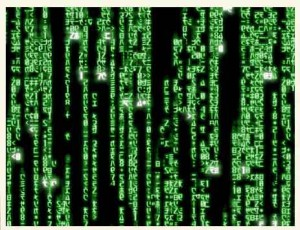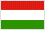# Month: May 2010

Suppose that $X_1,\ldots,X_n$ and $Y_1,\ldots,Y_n$ are two independent samples following a Gaussian law on $\mathbb{R}^p$ with zero mean and unknown invertible covariances $A$ and $B$. We consider the situation where $p$ is much larger than $n$ but we assume that $A^{-1}$ and $B^{-1}$ are sparse (conditional independence on the components). How can we test efficiently if $A=B$? Same question if the sparsity structure is assumed in $A$ and $B$ rather than on their inverse (independence instead of conditional independence).

Suppose that $(X_1,Y_1),\ldots,(X_n,Y_n)$ is a sample drawn from some unknown law on $\mathbb{R}^2.$ How can we efficiently test if the marginal laws are identical? More generally, suppose that $\ldots,Z_{-1}, Z_0, Z_1,\ldots$ is a time series. How can we test if the series is stationary? In other words, how can we test a nonparametric linear structure from the observation of a sample of an unknown law? Random projections?

For these questions coming from applications, we seek for a concrete usable answer…

This post is inspired from (separate) informal discussions with Georges Oppenheim and Didier Concordet. My friend and colleague Christophe Giraud told me that he is working with Nicolas Verzelen and Fanny Villers on the first question.

Last Updated on 2019-11-10I am not fond of the large deviations industry, but I like very much the Sanov theorem associated to the law of large numbers expressed on empirical distributions. The rate function in this large deviation principle is the Kullback-Leibler relative entropy.  It turns out that a very pleasant Sanov type theorem exists for certain models of random matrices. Namely, let $V:\mathbb{R}\to\mathbb{R}$ be a continuous function such that $\exp(-V)$ is Lebesgue integrable and  $V$ grows faster than the logarithm at infinity. For instance, one may take $V(x)=x^2/2$. Let $H_n$ be a random $n\times n$ Hermitian matrix with Lebesgue density proportional to

$$H\mapsto \exp\left(-n\mathrm{Tr}(V(H))\right).$$

Let $\lambda_{n,1},\ldots,\lambda_{n,n}$ be the eigenvalues of $H_n$. Their ordering  does not matter here. These eigenvalues are real since $H_n$ is Hermitian.  The unitary invariance of the law of $H_n$ allows to show that the law of $\lambda_{n,1},\ldots,\lambda_{n,n}$ , quotiented by the action of the symmetric group, has Lebesgue density proportional to

$$(\lambda_{n,1},\ldots,\lambda_{n,n})\mapsto \exp\left(-\sum_{k=1}^n nV(\lambda_{n,k})\right)\prod_{i\neq j}|\lambda_{n,i}-\lambda_{n,j}|^2$$

which can be rewritten as

$$(\lambda_{n,1},\ldots,\lambda_{n,n})\mapsto \exp\left(-\sum_{k=1}^n nV(\lambda_{n,k})+\frac{1}{2}\sum_{i\neq j}\log|\lambda_{n,i}-\lambda_{n,j}|\right).$$

The Vandermonde determinant comes from the Jacobian of the diagonalization seen as a change of variable (integration over the eigenvectors), and can also be seen as the discriminant (resultant of $P, P’$) of the characteristic polynomial $P$. Let us consider the random counting probability measure of these eigenvalues:

$$L_n:=\frac{1}{n}\sum_{k=1}^n\delta_{\lambda_{n,k}}$$

which is a random element of the convex set $\mathcal{P}$ of probability measures on $\mathbb{R}$. A very nice result due to Ben Arous and Guionnet states that for the topology of the narrow convergence, the sequence $(L_n)_{n\geq1}$ satisfies to a large deviation principle with speed $n^2$ and good rate function $\Phi$ given up to an additive constant by

$$\mu\in\mathcal{P}\mapsto \Phi(\mu):=\int\!V\,d\mu-\int\!\int\!\log|x-y|\,d\mu(x)d\mu(y).$$

In other words, we have for every nice set $A\subset\mathcal{P}$, as $n\to\infty$,

$$\mathbb{P}(L_n\in A)\approx \exp\left(-n^2\inf_{\mu\in A}\Phi(\mu)\right).$$

The second term in the definition of $\Phi(\mu)$ is the logarithmic energy of $\mu$ (minus the Voiculescu free entropy). When $V(x)=x^2/2$, then $H_n$ belongs to the Gaussian Unitary Ensemble and  the Wigner semicircle law is a maximum of this entropy under a second moment constraint, see e.g. the book by Saff and Totik. In particular, since the proof does not involve the underlying almost sure convergence theorem (in contrary to the Cramer theorem which involves the law of large numbers), the large deviation principle of Ben Arous and Guionnet yields via the first Borel-Cantelli lemma a new proof of the Wigner theorem.

In my opinion (I have a mathematical physicist soul!) the large deviation principle of Ben Arous and Guionnet is one of the nicest results in random matrix theory. It explains the appearance of the Wigner semicircle law in the Wigner theorem via a maximum entropy or minimum energy under moments constraints. Unfortunately, the result is only available for unitary invariant ensembles and does not cover the case of non Gaussian Wigner matrices with i.i.d. entries, for which the Wigner theorem is still valid. It is tempting to seek for a version of this large deviation principle for such non Gaussian Wigner matrices. The answer is not known. It is not clear that the sole finite positive variance assumption is enough to ensure that the rate function is the one of the Gaussian Unitary Ensemble. It is probable that the rate function will depend on the law of the entries. However, the arg-infimum of this function is still the Wigner semicircle law.

The proof of Ben Arous and Guionnet relies crucially on the explicit knowledge of the law of the spectrum, due to the unitary invariance of the model (roughly, if one puts the Vandermonde determinant into the exponential as a potential, it looks like a discrete Voiculescu entropy). Ben Arous and Zeitouni have used essentially the same method in order to establish a large deviation principle for the non-Hermitian version of the model, yielding a new proof of the circular law theorem for the Ginibre Ensemble.

It could be nice to connect these large deviations principles with transport inequalities. For connections between these two universes, take a look at the recent survey by Gozlan and Leonard.

Last Updated on 2019-11-10

Ma modeste expérience personnelle à Météo-France, aux Universités de Toulouse, d’Oxford,  et de Paris-Est Marne-la-Vallée, ainsi qu’à l’INRA, m’incite à penser qu’il ne faut pas opposer science fondamentale et appliquée. Nombre de tensions proviennent du petit nombre de ceux qui ont mené ces deux activités dans leur vie scientifique. Il m’avait fallu, pendant ma thèse, admettre  à contre cœur que les mathématiciens sont bien plus fragmentés et segmentés que les mathématiques elles-mêmes. Quelques années supplémentaires dans le monde de la recherche m’ont conduit à poursuivre cette réflexion sur ces humains qui font la science.

Il y a par exemple chez certains mathématiciens, la tentation d’apposer un verni appliqué sans se soucier vraiment des applications réelles, et chez certains scientifiques non mathématiciens, une recherche de respectabilité par la mathématisation sans se soucier de sa pertinence. Ces deux attitudes sont de plus parfaitement compatibles et peuvent constituer le socle de collaborations assez productives surfant sur les modes du moment. Cette sorte de mythe du quantitatif est peut-être le prix à payer pour faire émerger parfois des interactions interdisciplinaires fécondes. Parallèlement, le mathématicien fondamental ordinaire méprise les applications et réduit les mathématiques appliquées à de la technologie ou à de l’ingénierie. Ce mépris du concret est avant tout préjudiciable aux mathématiques. On ne se grandit pas en méprisant autrui, mais plutôt en tentant de faire mieux. Les Donoho, Lax, Meyer, et Tao, petits ou grands, semblent bien rares.

Au delà de l’utilitarisme primaire, se trouve une attitude qui consiste à toujours s’intéresser à des problèmes concrets, et à ne développer de théorie abstraite qu’au travers de cette motivation. C’est par exemple ce que pratiquent si souvent Aldous et Diaconis, parmi d’autres. Cela nécessite une certaine ouverture d’esprit, de la curiosité, et tente d’éviter le développement d’abstract non-sense dans les mathématiques appliquées.

Il faut cependant tenir compte d’aspects sociologiques supplémentaires. Les domaines scientifiques sont si vastes aujourd’hui que les chercheurs sont fortement poussés à l’hyper-spécialisation. Rares sont ceux qui sont à la fois généralistes et spécialistes dans un domaine. Il est moins risqué et plus efficace de mettre en œuvre un nombre limité de techniques bien maîtrisées dans un cadre précis pendant de nombreuses années. Un chercheur agissant de la sorte ne sortira que rarement de son moule doctoral ou post-doctoral. Cela constitue presque la règle aujourd’hui. Cependant, le système actuel est malgré tout assez souple pour tolérer toutes sortes d’écarts au modèle dominant. Cela m’est bien agréable car j’aurais sans doute renoncé à ce métier s’il me condamnait, à cause de mes limites, à une forme de fermeture, à une optimisation coût-bénéfice trop éloignée de ma manière de penser. Je dois dire que rencontrer mes semblables m’a beaucoup apporté.

Au bout du compte, sur le plan humain, c’est le plaisir à exercer son métier qui compte le plus, tandis que sur le plan scientifique, c’est la (relative) qualité de la production qui importe. Mais tout cela n’est-il pas que l’expression de la comédie humaine ? Au delà des activités dérisoires de chacun, la production scientifique globale subit une lente digestion collective, et l’Histoire fait son tri implacable. Combien avez-vous lu d’articles originaux de Kolmogorov ?

La comédie humaine est pleine d’enseignements, bien que celle qui se joue au moment des recrutements ait un rapport assez curieux à l’enseignement. Idéalement, un recrutement devrait étoffer le groupe sur le plan scientifique, le dynamiser, l’ouvrir, tout en évitant les catastrophes pédagogiques. Cet idéal semble assez peu compatible avec le micro-étiquetage des profils de postes pratiqué ici et là. Il faut dire que l’absence d’étiquetage n’est envisageable que si le groupe est raisonnable et ne donne pas dans l’ostracisme. Dans cette comédie où les destins se jouent, recruter meilleur ou différent fait parfois peur. La hiérarchie intra et inter-disciplinaire s’exprime aussi, et les plus puissants ne sont pas toujours les plus ouverts. Malgré tout, certains groupes parviennent à faire preuve de raison et d’audace, et en sont récompensés sur le long terme. J’aime à penser que les choses ne peuvent changer, lentement, que si certains font tout pour qu’elles changent rapidement. Ce paradoxe de l’engagement, qui fait fondre les illusions, encourage pourtant à agir inlassablement.

Note : ce texte est tiré de l’avant-propos de mon mémoire d’habilitation à diriger des recherches (2008).

Last Updated on 2021-04-01The strength and beauty of the Hungarian mathematical school is  quite fascinating.  Everybody knows Paul Erdős, but many mathematicians ignore that great minds such as Eugene Wigner and John von Neumann were actually Hungarian (schoolmates in Budapest and colleagues in Princeton). During the twentieth century, Budapest and Szeged were important cultural and scientific centers for Central and Eastern Europe, with influences from Germany and Russia. I maintain a list of Hungarian mathematicians. Comments are welcome!

Digression: the random matrices of Wigner and the operators algebras of von Neumann (beware that their works are not resctricted to these subjects!) were connected by the free probability theory of Voiculescu, a Romanian mathematician, in the late twentieth century.

Last Updated on 2010-08-22

Syntax · Style · .